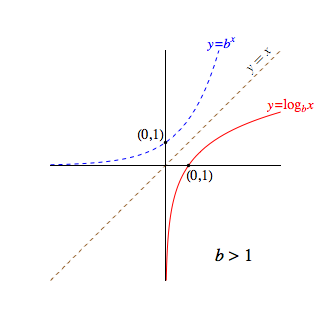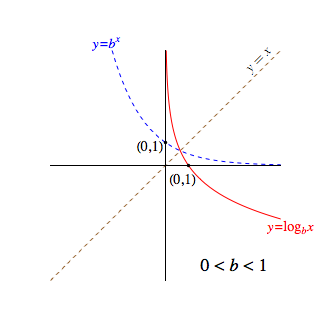Logarithmic Functions

Since every exponential function $f(x) = b^x$, where $b \gt 0, b \neq 1$, passes the horizontal line test, each such function must be invertible.

Let us refer to the inverse of $f$ as the logarithm base $b$ of $x$. Further, let us denote this function $f$ by $\log_b x$, leaving off the parentheses around the input $x$ unless they are needed. Notice, this mimics the style in which we write trigonometric functions.

Of course, by virtue of being $f$'s inverse, we know that

$$y=\log_b x \quad \textrm{if and only if} \quad b^y=x$$

In the special case when $b=e$, we write $y=\ln x$, saying that $y$ is the natural logarithm of $x$. This then likewise requires

$$y = \ln x \quad \textrm{if and only if} \quad e^y=x$$

Recalling that the graph of an inverse function is found by reflecting the original function over the line $y=x$, we discover that the graphical nature of a logarithmic function also depends largely on whether the base $b$ is greater than or less than one, as the graphs below suggest.Several properties of log functions are immediately evident from both the above graphs, such as:

• The domain consists of only positive real values, $(0,\infty)$

• The range is the set of all reals, $\mathbb{R}$

• The only intercept is $(1,0)$

• The line $x=0$ (i.e., the $y$-axis) is a vertical asymptote

• The function increases on its entire domain if $b \gt 1$, and decreases on the same if $0 \lt b \lt 1$

• The function is one-to-one

Other properties follow immediately from the inverse relationship with a related exponential function:

$$\begin{array}{rcll} \log_b b &=& 1 & \scriptsize{\textrm{since } b^1 = b}\\ \log_b 1 &=& 0 & \scriptsize{\textrm{since } b^0 = 1}\\ \log_b b^x &=& x &\scriptsize{\textrm{since for inverse functions } f \textrm{ and } f^{-1} \textrm{ we know that } f^{-1}(f(x)) = x}\\ b^{\log_b x} &=& x & \scriptsize{\textrm{since for inverse functions } f \textrm{ and } f^{-1} \textrm{ we know that } f(f^{-1}(x)) = x} \end{array}$$

Of course, in the special case where the base is $e$, the above four properties take the following forms:

$$\begin{array}{rcl} \ln e &=& 1\\ \ln 1 &=& 0\\ \ln e^x &=& x\\ e^{\ln x} &=& x \end{array}$$

Indeed, the inverse relationship between logarithmic and exponential functions allows us to write any equation in the exponential form $b^y=x$ in an equivalent logarithmic form, and vice-versa, as shown below:

$$\begin{array}{rclcrcl} \log_3 9 &=& 2 &\longleftrightarrow& 3^2 &=& 9\\ \log_{10} 0.00001 &=& -5 &\longleftrightarrow& 10^{-5} &=& 0.00001\\ \log_8 4 &=& \frac{2}{3} &\longleftrightarrow& 8^{2/3} &=& 4\\ \log_{1/2} 4 &=& -2 &\longleftrightarrow& \left( \frac{1}{2} \right)^{-2} &=& 4\\ \log_b \sqrt{b} &=& \frac{1}{2} &\longleftrightarrow& b^{1/2} &=& \sqrt{b}\\ \ln 1 &=& 0 &\longleftrightarrow& e^0 &=& 1\\ \log_3 c &=& N &\longleftrightarrow& 3^N &=& c\\ \ln 4 &=& x &\longleftrightarrow& e^x &=& 4 \end{array}$$

As the above demonstrate you can think of $\log_b x$ as "the exponent that must be placed on $b$ to produce $x$". Remembering this phrase can often make evaluating logarithms quick and easy to do in one's head.

Taking this idea a step further, if logarithms are exponents as the phrase above suggests, then the laws of exponents will some force analogous laws for logarithms.

Three such laws/properties for logarithms are given below. (Click the links provided to see proofs of each.)

• $\displaystyle{\log_b xy = \log_b x + \log_b y}$

First note that we can evaluate $\log_b x$ and $\log_b y$, since $x,y,$ and $b$ are positive real values with $b \neq 1$. Let us denote these two values by $m$ and $n$, so that

$$\log_b x = m \quad \textrm{and} \quad \log_b y = n$$

If we express the above in exponential form, we have

$$b^m = x \quad \textrm{and} \quad b^n = y$$

With the above, the rest of the proof follows quickly, as shown below. Notice how the proof hinges on the fact that $b^m b^n = b^{m+n}$, one of our laws of exponents.

$$\begin{array}{rcl} \log_b xy &=& \log_b b^m b^n \\ &=& \log_b b^{m+n}\\ &=& m + n\\ &=& \log_b x + \log_b y \end{array}$$

• $\displaystyle{\log_b \frac{x}{y} = \log_b x - \log_b y}$

First note that we can evaluate $\log_b x$ and $\log_b y$, since $x,y,$ and $b$ are positive real values with $b \neq 1$. Let us denote these two values by $m$ and $n$, so that

$$\log_b x = m \quad \textrm{and} \quad \log_b y = n$$

If we express the above in exponential form, we have

$$b^m = x \quad \textrm{and} \quad b^n = y$$

With the above, the rest of the proof follows quickly, as shown below. Notice how the proof hinges on the fact that $\displaystyle{\frac{b^m}{b^n} = b^{m-n}}$, one of our laws of exponents.

$$\begin{array}{rcl} \log_b \frac{x}{y} &=& \log_b \frac{b^m}{b^n}\\ &=& \log_b b^{m-n}\\ &=& m - n\\ &=& \log_b x - \log_b y \end{array}$$

• $\displaystyle{\log_b x^n = n \log_b x}$

First note that we can evaluate $\log_b x$, since $x$ and $b$ are positive real values with $b \neq 1$. Let us denote this value by $m$, so

$$\log_b x = m$$

Expressing the above in exponential form, we then have $$b^m = x$$

Now the rest of the proof follows quickly, as shown below. Notice how the proof hinges on the fact that $(b^m)^n = b^{mn}$, one of our laws of exponents.

$$\begin{array}{rcl} \log_b x^n &=& \log_b (b^m)^n \\ &=& \log_b b^{mn}\\ &=& mn\\ &=& n\log_b x \end{array}$$

Another very useful property of logarithms derived from the laws of exponents is the Change of Base Theorem, shown below. This theorem allows one to rewrite any logarithm (say in base $y$) in terms of logarithms involving any desired legal base $b$ that one wishes.

$\displaystyle{\log_y x = \frac{\log_b x}{\log_b y}}$

First note that we can evaluate $\log_b x, \log_b y$ and $\log_y x$, since $x,y,$ and $b$ are all positive real values with $b,y \neq 1$. Let us denote the last two values by $m$ and $n$, so that

$$\begin{array}{rcl} \log_b y &=& m\\ \log_y x &=& n\\ \end{array}$$

If we express the above in exponential form, we have

$$\begin{array}{rcl} b^m &=& y\\ y^n &=& x\\ \end{array}$$

Note, that we know from the laws of exponents that

$$b^{mn} = (b^m)^n = y^n = x$$

Rewriting the resulting $b^{mn} = x$ in logarithmic form, we have

$$\log_b x = mn$$

Now, we can substitute for $m$ and $n$ to obtain

$$\log_b x = (\log_b y)(\log_y x)$$

Finally, dividing both sides by $\log_b y$ completes the proof.

$$\frac{\log_b x}{\log_b y} = \log_y x$$